# Extensions to the Grammar of Graphics

## Preliminaries

We load all the packages used in the examples, if installed, and set flags to skip examples if they are not available.

library(ggpp)
library(tibble)
library(dplyr)
eval_ggrepel <- requireNamespace("ggrepel", quietly = TRUE) &&
packageVersion("ggrepel") > "0.9.1"
if (eval_ggrepel) library(ggrepel)
eval_magick <- requireNamespace("magick", quietly = TRUE)

As we will use text and labels on the plotting area we change the default theme to an uncluttered one.

old_theme <- theme_set(theme_bw())

# Introduction to the extensions

## Data labels, annotations and insets

Data labels add textual information directly related to individual data points (shown as glyphs). Text position in this case is dependent on the scales used to represent data points. Text is usually displaced so that it does not occlude the glyph representing the data point and when the link to the data point is unclear, this link is signaled with a line segment or arrow. Data labels are distinct from annotations but instead contribute to the representation of data on a plot or map.

References are lines, shading or marks used to help the reading of a plot. These elements are used to highlight specific values on an axis or a region in a plot. They, like data labels, are positioned relative to the scales used for data. The position of data labels and lines, glyphs or shading used as reference cannot be altered by the designer of a plot, as the position conveys information. I will use the term data labels irrespective if the “labels” are textual or graphical, like icons and small plots and simple tables linked to data points or map features.

According to Koponen and Hildén (2019), in a statistical chart “annotations can be used to draw reader attention to relevant detail”. These authors use as an example a text box in a plot to highlight a data point that is off-scale and has been “squeezed” to a position immediately outside the plotting area.

Annotations differ from data labels, in that their position is decoupled from their meaning. Insets can be thought as larger, but still self-contained annotations. In most cases the reading of inset tables and plots depends only weakly on the plot or map in which they are included.

In the case of annotations and insets the designer of a data visualization has the freedom to locate them anywhere, as long they do not occlude features used to describe data. I will use the term annotation irrespective if the “labels” are textual or graphical. Insets are similar to annotations, but the term inset is used when an annotation’s graphical or textual element is complex and occupies more space within the plotting area. Insets can be moved from within the main plotting area to being adjacent to it, e.g., as a smaller panel, without any loss of meaning .

That the position of annotations and insets is independent of the plotted data cannot be expressed using the grammar of graphics (GG) as implemented in package ‘ggplot2’.

## Annotations in ‘ggplot2’

The plotting of data using package ‘ggplot2’ is described by Wickham (2016) and several other books. Chapter 7 in Aphalo (2020) not only describes the grammar used by ‘ggplot2’ but also several extensions to it, including those provided by packages ‘ggpp’ and ‘ggpmisc’.

In The Layered Grammar of Graphics (Wickham 2000) as implemented in package ‘ggplot2’ (Wickham 2016) annotations are “second class” features. As layers they behave differently than data layers: Only constant values can be mapped to aesthetics and do no support faceting into panels. In essence annotate() disconnects the resulting plot elements from the data source and faceting, but not from the scales used to graphically display the data values.

From the data visualization perspective the main practical and conceptual difference between data labels and annotations is in the scales used to position them within the plotting area. Instead of using an annotate() function that deviates from the grammar of graphics to implement annotations, we could retain the use of the grammar of graphics for annotations but add support of native plot coordinates (npc). Support of annotations done in this way would allow “annotation layers” to behave almost identically to “data layers”, and use the same grammar. The x and y position aesthetics used for data could be supplemented with pseudo-aesthetics without any translation relative to the native plotting coordinates of the plotting area or viewport. Doing so, would allow the graphic design flexibility conceptually inherent to annotations within a user-friendly syntax.

## Extending ‘ggplot2’

Based on this insight, a new approach to adding annotations and insets was implemented in package ‘ggpmisc’ (>= 0.3.1) through two new x and y pseudo-aesthetics, npcx and npcy, and corresponding dumb scales and various geometries that make use of them. These scales and geometries were implemented as an extension to package ‘ggplot2’ and are in package ‘ggpp’.

Before showing how this works in practice, we load the package and set an uncluttered theme as default.

library(ggpp)
theme_set(theme_bw() + theme(panel.grid = element_blank()))

Native plot coordinates have range 0..1. However, the “npc” geoms also recognize some positions by name with the same character strings as used for text justification in ‘ggplot2’. The default justification is "inward", as this protects from clipping at the edges the plotting area irrespective the arguments passed to the witdth and height parameters of R’s graphic devices.

p1 <- ggplot(mtcars, aes(factor(cyl), mpg)) +
geom_point() +
geom_text_npc(data = data.frame(x = c("left", "left"),
y = c("top", "bottom"),
label = c("Most\nefficient",
"Least\nefficient")),
mapping = aes(npcx = x, npcy = y, label = label),
size = 3)
p1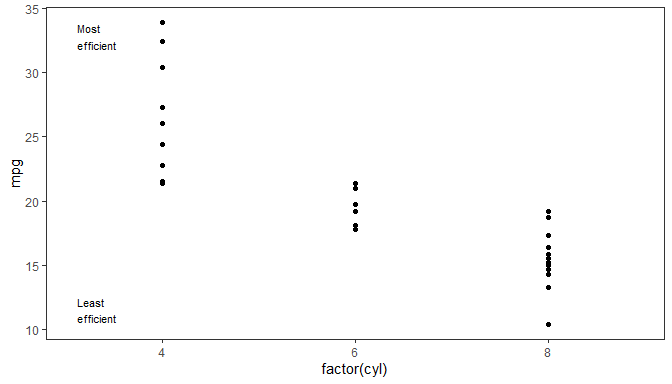The advantage of this approach becomes apparent when the limits of the y scale are unknown or vary. When a script or user defined plotting function sets the scale limits based on the input data, in the absence of the extensions proposed here, setting annotations consistently within the plotting area becomes laborious. The example below, shows how the annotations remain at the desired position when the y scale limits are expanded.

p1 + expand_limits(y = 0)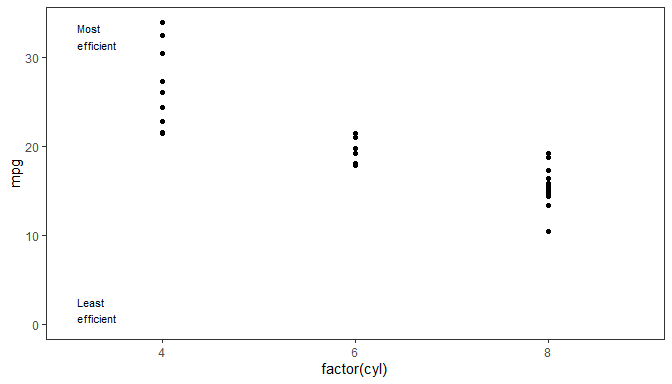To support the existing syntax for annotations using the new geometries, function ggplot2::annotate() is overridden when package ‘ggpp’ is loaded. The new definition adds support for the new pseudo-aesthetics npcx and npcy retaining its original ‘ggplot2’ behaviour in all other respects.

ggplot(mtcars, aes(factor(cyl), mpg)) +
geom_point() +
annotate(geom = "text_npc",
npcx = c("left", "left"),
npcy = c("top", "bottom"),
label = c("Most\nefficient",
"Least\nefficient"),
size = 3)Inset plots can be added with the same syntax using geom_plot_npc(). They can be thought also as being annotations. Here we use annotate() but geom_plot() can be also used directly, in which case the inset plots can be different for each panel.

p2 <- ggplot(mtcars, aes(factor(cyl), mpg, colour = factor(cyl))) +
stat_boxplot() +
labs(y = NULL) +
theme_bw(9) +
theme(legend.position = "none",
panel.grid = element_blank())

ggplot(mtcars, aes(wt, mpg, colour = factor(cyl))) +
geom_point() +
annotate("plot_npc", npcx = "left", npcy = "bottom", label = p2) +
expand_limits(y = 0, x = 0)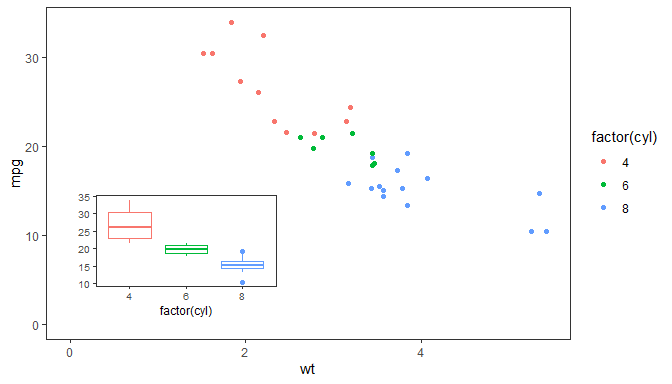A simple example with facets, labelling of panels in a traditional way as required by some book and journal styles. In this case panel tags are added within the plotting area at a consistent “npc” location with free scale limits in panels.

ggplot(mtcars, aes(wt, mpg)) +
geom_point() +
geom_text_npc(data = data.frame(cyl = levels(factor(mtcars$cyl)), label = LETTERS[seq_along(levels(factor(mtcars$cyl)))],
x = 0.90,
y = 0.95),
mapping = aes(npcx = x, npcy = y, label = label),
size = 4) +
facet_wrap(~factor(cyl), scales = "free") +
theme(strip.background = element_blank(),
strip.text = element_blank())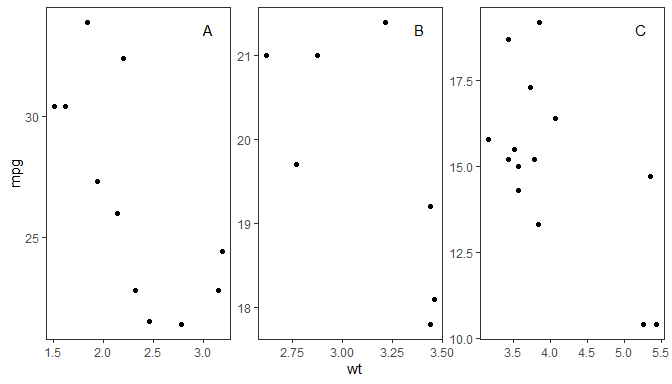This approach was first implemented in ‘ggpmisc’ version 0.3.1 released in April 2919. The implementation now in package ‘ggpp’ can be considered stable. However, this implementation is to an extent dependent on undocumented behaviour of ‘ggplot2’ functions, which means that future updates to ‘ggplot2’ could break this functionality.

## When to use annotations and when insets

Package ‘ggpmisc’ adds support for various plot annotations and reference guides based on model fits and other statistics. It also adds support for some data labels related to data features. While developing these statistics in ‘ggpmisc’, it became clear that expanding the grammar of graphics’s support for annotations would simplify the new code considerably and also more cleanly separate the computations on data from the positioning of annotations. These extensions to the grammar are now in ‘ggpp’.

Table. Geometries useful for data labels and annotations. Currently implemented ordinary geometries and their npc versions. The rightmost column shows the expected class of the objects mapped to the label aesthetic. When using annotate() to add a single plot, table or grob as an inset, enclosing them in a list is allowed, but not a requirement. All those geoms whose names are highlighted in italics support plotting of connecting segments or arrows when a the coordinates have been modified by a position function.

Geometries
Data labels Annotations (npc) label aes.
ggplot2::geom_text() geom_text_npc() character
ggplot2::geom_label() geom_label_npc() character
ggrepel::geom_text_repel() character
ggrepel::geom_label_repel() character
geom_text_s() character
geom_label_s() character
geom_point_s()
geom_plot() geom_plot_npc() list(<ggplot>)
geom_table() geom_table_npc() list(<data.frame>)
geom_grob() geom_grob_npc() list(<grob>)
geom_img() (planned) geom_img_npc() list(<raster>)

When adding an informative element to a plot, assess whether it is an annotation or a data label. To decide on the best approach, consider if the location of the element is more “naturally” expressed in the original data units or as position relative to the edges or centre of the plotting area. In the second case, prefer the “npc” geoms as you are dealing with annotations, otherwise, use the ordinary geometries as you are dealing with data labels or data points.

## Positioning data labels

Nudging is normally used to displace text labels so that they do not overlap the points or lines representing the data being labelled. Two enhanced versions of position_nudge() are provided, position_nudge_center() and position_nudge_line(). These functions make it possible to apply nudging that varies automatically according to the relative position of points with respect to arbitrary points or lines, or with respect to a polynomial or smoothing spline fitted on-the-fly to the the observations.

A limitation of ‘ggplot2’ is that only one position function can be used in a layer. This makes it difficult to add text labels to stacked or dodged bars or columns, or the labelling of points that have been jittered. The solution provided by ‘ggpp’ are wrappers on these position functions that add support for nudging.

All these new position functions, instead of deleting the original positions, they rename the variables in data containing these coordinates. This makes it possible for geometries to draw connecting segments or arrows between old and new positions. These functions are backwards compatible and can be used in place of those from ‘ggplot2’. The geometries from ‘ggrepel’ (> 0.9.1) and many of those from ‘ggpp’ can make use of the original coordinates kept in data.

Position functions
Position (origin kept) Position (origin discarded)
position_nudge_keep() ggplot2::position_nudge()
ggrepel::postion_nudge_repel() ggplot2::position_nudge()
position_nudge_centre()
position_nudge_line()
position_jitter_keep() ggplot2::position_jitter()
position_dodge_keep() ggplot2::position_dodge()
position_dodge2_keep() ggplot2::position_dodge2()
position_stack_keep() ggplot2::position_stack()
position_fill_keep() ggplot2::position_fill()
position_jitterdodge_keep() (planned) ggplot2::position_jitterdodge()
position_jitternudge()
position_dodgenudge()
position_dodge2nudge()
position_stacknudge()
position_fillnudge()
position_jitterdodgenudge() (planned)

## Highlighting data features

If maxima or minima are to be labelled in a plot, this can be automated so that the feature and its labelling is automated. This is possible with stat_peaks() and stat_valleys(). Marginal annotations with symbols and vertical and horizontal lines can be used to highlight summary values or events/conditions. The geoms geom_quadrant_lines(), geom_vhlines(), geom_x_margin_arrow(), geom_x_margin_grob(), geom_x_margin_point(), geom_y_margin_arrow(), geom_y_margin_grob() and geom_y_margin_point() make such labelling easy.

## Local density of observations

There are two aspects to this: highlighting with colours dense or sparse regions, or using density to decide which observations to label. In this cases we can use stat_dens1d_labels() and stat_dens2d_labels(), stat_dens1d_filter() and stat_dens2d_filter(), and stat_dens1d_filter_g() and stat_dens2d_filter_g().

## Summary statistics

With some types of data it is common to compute a summary per quadrant, rather than based on a prior grouping. The stat stat_quadrant_counts() can de used to annotate plots with the number of observations in each quadrant. stat_summary_xy() is similar to stat_summary() from ‘ggplot2’ but useful when both x and y are continuous variables. It allows the use the same, or different functions to summarise the data over x and y. In contrast stat_centroid() always applies the same function over x and y.

## ggplot methods

Being ggplot() defined as a generic method in ‘ggplot2’ makes it possible to define specializations, and we provide two for time series stored in objects of classes ts and xts which automatically convert these objects into tibbles and set by default the aesthetic mappings for x and y automatically. A companion function try_tibble() is also exported.

## Thoughts for the future

Although we have discussed data independent scales only for x and y aesthetics, the same consideration applies to other aesthetics like colour. It seems generally useful to allow aesthetics to use a different scale for annotations than for data. Defining pseudo-aesthetics for annotations instead of allowing multiple scales for each aesthetic in a plot would add flexibility while still keeping the guarantee that the meaning of aesthetics’ values remains consistent across all plot elements representing data.

## List of references

Aphalo, Pedro J. (2020) Learn R: As a Language. The R Series. Boca Raton and London: Chapman and Hall/CRC Press. ISBN: 978-0-367-18253-3. 350 pp.

Koponen, J; Hildén, J. (2019) Data visualization handbook. Aalto ARTS books, Espoo. ISBN 978-952-60-7449-8.

Wickham H. (2010) A Layered Grammar of Graphics. Journal of Computational and Graphical Statistics 19: 3–28.

Wickham H. (2016) ggplot2: Elegant Graphics for Data Analysis. Springer International Publishing. ISBN 978-3-319-24275-0.

# Examples

Some of the examples below are evaluated only if packages ‘ggrepel’, and ‘magick’ are available.

## ggplot methods

ggplot() methods for classes "ts" and "xts" automate plotting of time series data, as x and y aesthetics are mapped to time and the variable of the time series, respectively. For plotting time series data stored in objects of other classes, see the conversion functions try_tibble() and try_data_frame() in the last section of this vignette.

By default, time is of class Date or of class POSIXct depending on how time is stored in the time series object. On-the-fly coversion of time to numeric is also possible (shown in the second example below).

class(lynx)
##  "ts"
ggplot(lynx) + geom_line()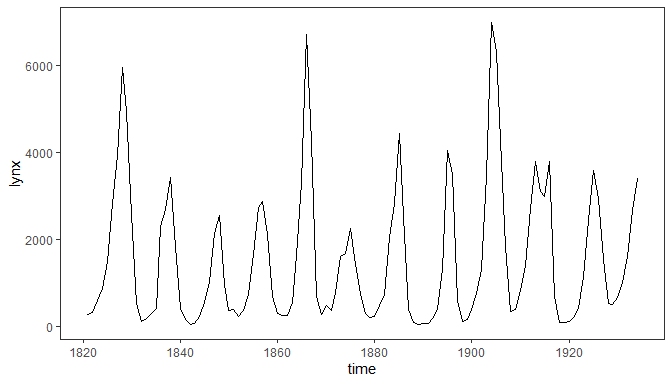The class of variable time, mapped to the x aesthetic, affects the scale used by default as well as the formatting of values when converted to character strings or printed. Here we force its conversion to numeric.

ggplot(lynx, as.numeric = FALSE) + geom_line()## Geometries

Three of the geometries described below allow the addition of plot layers containing insets. Insets can be plots, tables, bitmaps, or grid objects. Insets can be also added as annotations. Using for data a tibble with a list column containing data frames or tibbles allows like any other geom, the use of grouping, multiple insets per panel, faceting with different tables per panel, and different number of insets in each panel, i.e., individual tables added to a plot with geom_table behave similarly to individual character values added with geom_text.

Other geometries also described in this section support the use of native plot coordinates for positioning elements in the plotting area. Obviously these geometries are not meant to be used to display data, but instead they make it possible to add annotations to plots consistently across data sets even when using scales with varying limits.

### geom_table() and stat_fmt_table()

The geometry geom_table() plots a data frame or tibble, nested in a tibble passed as data argument, using aesthetics x and y for positioning, and label for the list of data frames containing the data for the tables. The tables are created as ‘grid’ grobs and added as usual to the ggplot object. In contrast to “standard” geoms, this geom by default does not inherit the globally mapped aesthetics. Tables are always added at their native sizes, which can be altered by changing the size of the text in them.

tb <- mpg %>%
group_by(cyl) %>%
summarise(hwy = median(hwy), cty = median(cty))

data.tb <- tibble(x = 7, y = 44, tb = list(tb))

ggplot(mpg, aes(displ, hwy, colour = factor(cyl))) +
geom_table(data = data.tb, aes(x, y, label = tb)) +
geom_point()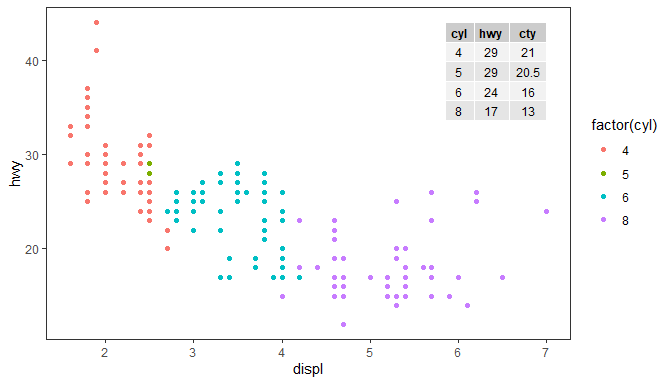In plots with a single panel it can be easier to use annotate() to add inset tables, giving the same plot as above. In this case single data frames, ggplots or grobs do not need to be wrapped in a list, although lists are also supported.

tb <- mpg %>%
group_by(cyl) %>%
summarise(hwy = median(hwy), cty = median(cty))

ggplot(mpg, aes(displ, hwy, colour = factor(cyl))) +
annotate("table", x = 7, y = 44, label = tb) +
geom_point() 

Table themes are supported through parameter table.theme and if variables or constants are mapped to the colour, fill, size, or family, aesthetics they override the corresponding default theme settings. The display of rownames and colnames can be enabled or disable through parameter table.rownames and table.colnames and the horizontal justification of text in the core of the table through parameter table.hjust.

Parameter table.theme accepts as arguments NULL for use of the current default, a ttheme constructor function such as those defined in package ‘gridExtra’, or the variations on them defined in this package. The active default can be set with function ttheme_set().

ggplot(mpg, aes(displ, hwy, colour = factor(cyl))) +
geom_table(data = data.tb, aes(x, y, label = tb),
table.theme = ttheme_gtsimple,
table.hjust = 0, colour = "darkred", fill = "#FFFFBB") +
geom_point()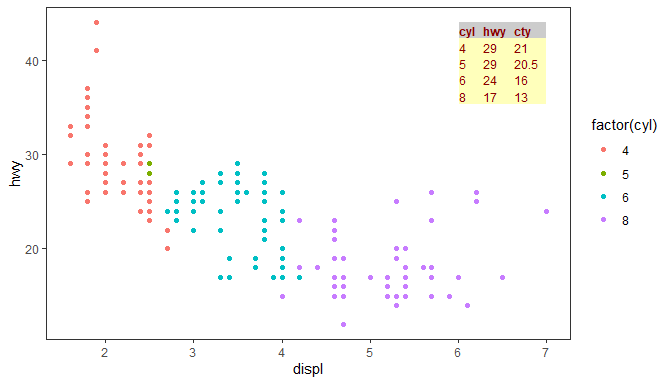Using stat_fmt_tb() we can rename columns and rows of the tibble, reorder them and/or select a subset of columns or rows as shown below. To provide a complete example we also replace the names of the scales for x, y and color aesthetics. Here we pass a character vector with the original names of the columns in full, but partial matching is tried when needed. It is also possible to use a numeric vector of positional indexes.

ggplot(mpg, aes(displ, hwy, colour = factor(cyl))) +
geom_table(data = data.tb, aes(x, y, label = tb),
table.theme = ttheme_gtlight,
size = 3, colour = "darkblue",
stat = "fmt_tb",
tb.vars = c(Cylinders = "cyl", MPG = "hwy"), # rename
tb.rows = 4:1) + # change order
labs(x = "Engine displacement (l)", y = "Fuel use efficiency (MPG)",
colour = "Engine cylinders\n(number)") +
geom_point() +
theme_bw()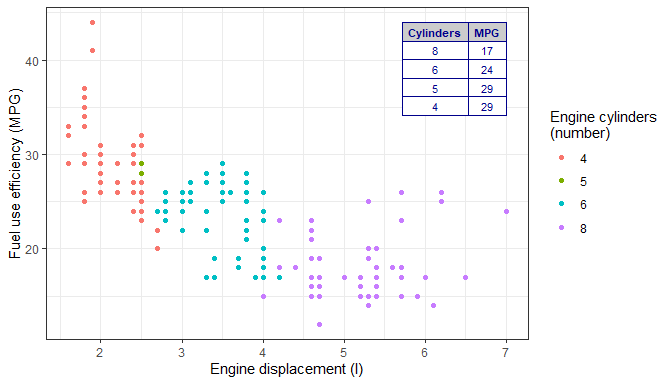Parsed text, using plot math syntax is supported in the table, with fall-back to plain text in case of parsing errors, on a cell by cell basis (see tableGrob() in package ‘gridExtra’ for details, as this function is used to build the table). Here we plot the MPG for city traffic and we can see that the plotting area expands to include the coordinates at which the table is anchored. Justification is by default set to "inward" which ensures that the table is fully within the plotting region.

tb.pm <- tibble(Parameter = c("frac(beta, a^2)", "frac(beta, a^3)"),
Value = c("10^2.4", "10^3.532"))
data.tb <- tibble(x = 7, y = 44, tb = list(tb.pm))
ggplot(mpg, aes(displ, cty)) +
geom_point() +
geom_table(data = data.tb, aes(x, y, label = tb), parse = TRUE) +
theme_bw()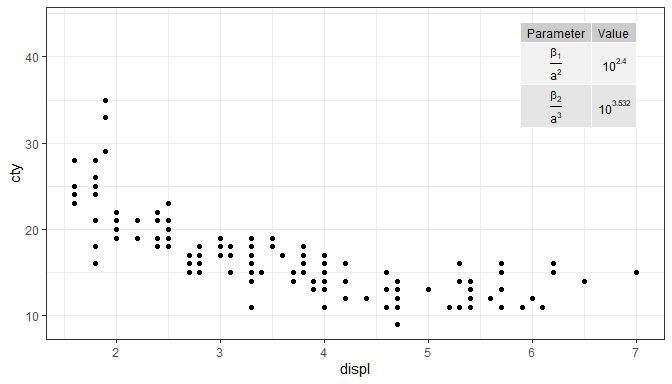As implemented, there is no limitation to the number of insets, and faceting is respected. If the base plot shows a map, multiple small tables could be superimposed on different countries or regions. The size of the table inset is given by the size aesthetic, so like for geom_text() it is independent of the ggplot2::theme() and controlled by the table.theme directly or indirectly through the size aesthetic.

Please see section Normalised Parent Coordinates below for a description of geom_table_npc().

### geom_plot()

The geom_plot() geometry plots ggplot objects, nested in a tibble passed as data argument, using aesthetics x and y for positioning, and label for the ggplot object containing the definition of the plot to be nested. With this approach in plots with facets the insets can be different in each panel. It is also possible to inset more than one plot in a single call simply by creating a tibble with multiple rows.

Behind the scenes, one Grob is created for each plot to be inset. The conversion is done with ggplotGrob() and the Grobs added to the main ggplot object.

As an example we produce a plot where the inset plot is a zoomed-in detail from the main plot. In this case the main and inset plots start as the same plot. In most cases the size of text and other elements in the inset should be smaller than in the main plot. Here we override the default theme setting the base_size from its default of 11 pt to 8 pt.

p <- ggplot(mpg, aes(displ, hwy, colour = factor(cyl))) +
geom_point()

data.tb <-
tibble(x = 7, y = 44,
plot = list(p +
coord_cartesian(xlim = c(4.9, 6.2),
ylim = c(13, 21)) +
labs(x = NULL, y = NULL) +
theme_bw(8) +
scale_colour_discrete(guide = "none")))

ggplot(mpg, aes(displ, hwy, colour = factor(cyl))) +
geom_plot(data = data.tb, aes(x, y, label = plot)) +
annotate(geom = "rect",
xmin = 4.9, xmax = 6.2, ymin = 13, ymax = 21,
linetype = "dotted", fill = NA, colour = "black") +
geom_point()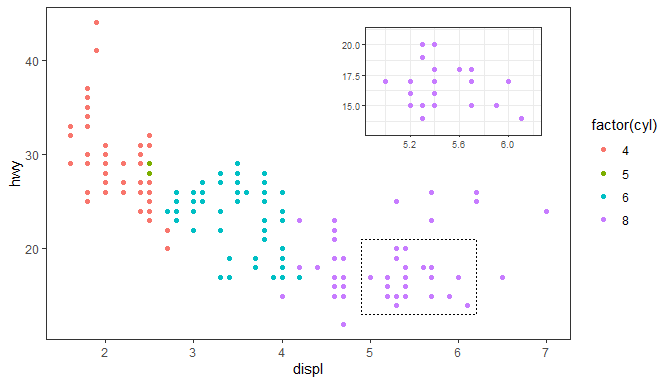In general, the inset plot can be any ggplot object, allowing the creation of very different combinations of main plot and inset plots. Here we use the inset to show summaries as in the previous example of an inset table.

p <- ggplot(mpg, aes(factor(cyl), hwy, fill = factor(cyl))) +
stat_summary(geom = "col", fun = mean, width = 2/3) +
labs(x = "Number of cylinders", y = NULL, title = "Means") +
scale_fill_discrete(guide = "none")

data.tb <- tibble(x = 7, y = 44,
plot = list(p +
theme_bw(8)))

ggplot(mpg, aes(displ, hwy, colour = factor(cyl))) +
geom_plot(data = data.tb, aes(x, y, label = plot)) +
geom_point() +
labs(x = "Engine displacement (l)", y = "Fuel use efficiency (MPG)",
colour = "Engine cylinders\n(number)") +
theme_bw()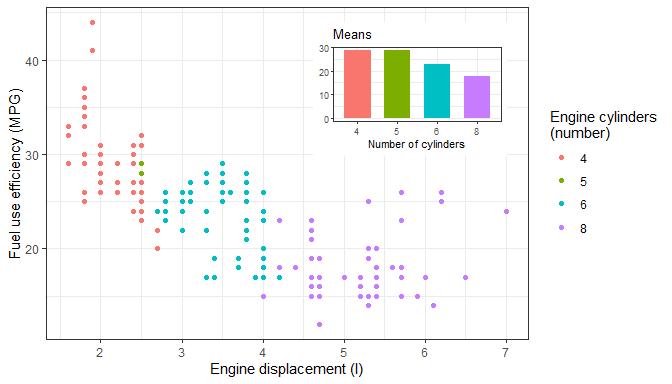The same plot as above can be created using annotate(), but be aware that when using facets in ‘ggplot2’ annotations are identical in all panels.

p <- ggplot(mpg, aes(factor(cyl), hwy, fill = factor(cyl))) +
stat_summary(geom = "col", fun = mean, width = 2/3) +
labs(x = "Number of cylinders", y = NULL, title = "Means") +
scale_fill_discrete(guide = "none")

ggplot(mpg, aes(displ, hwy, colour = factor(cyl))) +
annotate("plot", x = 7, y = 44, label = p + theme_bw(8)) +
geom_point() +
labs(x = "Engine displacement (l)", y = "Fuel use efficiency (MPG)",
colour = "Engine cylinders\n(number)") +
theme_bw()

As implemented, there is no limitation to the number of insets, and faceting is respected. If the base plot shows a map or a bitmap, multiple small plots could be superimposed on different countries or regions. The size of the insets is controlled by the vp.width and vp.height aesthetics as a fraction of the main plot’s plotting region. Consequently, the insets are scaled together with the main plot. A possible unintuitive but useful feature, is that the theme is linked to each plot.

Please see section Normalised Parent Coordinates below for a description of geom_plot_npc().

### geom_grob()

The geom_grob() geometry plots grobs (graphical objects as created with ‘grid’), nested in a tibble passed as data argument, using aesthetics x and y for positioning, and label for the Grob object. While geom_table() and geom_plot() take as values mapped to the label aesthetics tibbles or data frames, and ggplots, respectively, and convert them into Grobs, geom_grob() expects Grobs ready to be rendered. This means that any Grob created using ‘grid’ or its extenssions can be added as a data label to a ggplot.

file.name <-
system.file("extdata", "Isoquercitin.png",
package = "ggpp", mustWork = TRUE)
grobs.tb <- tibble(x = c(0, 10, 20, 40), y = c(4, 5, 6, 9),
width = c(0.05, 0.05, 0.01, 1),
height =  c(0.05, 0.05, 0.01, 0.3),
grob = list(grid::circleGrob(),
grid::rectGrob(),
grid::textGrob("I am a Grob"),
grid::rasterGrob(image = Isoquercitin)))

ggplot() +
geom_grob(data = grobs.tb,
aes(x, y, label = grob, vp.width = width, vp.height = height),
hjust = 0.7, vjust = 0.55) +
scale_y_continuous(expand = expansion(mult = 0.3, add = 0)) +
scale_x_continuous(expand = expansion(mult = 0.2, add = 0)) +
theme_bw(12)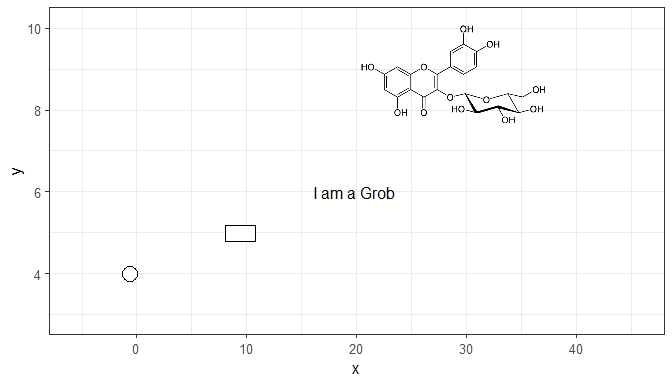As shown above for inset tables and inset plots, it is also possible to use annotate() with Grobs. The next example insets a single Grob. Here we reuse the bitmap Isoquercitin read in the previous example. The Grob is contained in a viewport. Here setting width = 1 (“npc” units) when creating the Grob from the bitmap ensures that the bitmap fills the width of the viewport (to ensure that the inset is not distorted, set only one of width or height). The argument to vp.width or vp.height, also in “npc” units, determines the size of the Grob relative to the size of the plotting area.

ggplot() +
annotate("grob", x = 1, y = 3, vp.width = 0.5,
label = grid::rasterGrob(image = Isoquercitin, width = 1)) +
theme_bw(12)

geom_grob() is designed thinking that its main use will in graphical annotations, although one could use it for infographics with multiple copies of each grob, this would go against the grammar of graphics. In this implementation grobs cannot be mapped to an aesthetic through a scale.

As implemented, there is no limitation to the number of insets and faceting is respected. If the base plot shows a map or a bitmap, multiple simple grobs (e.g. national flags) could be superimposed on different countries. The size of the insets is controlled by the vp.width and vp.height aesthetics as a fraction of the main plot’s plotting region. Consequently, the insets are scaled together with the main plot.

Please see section Normalised Parent Coordinates below for a description of geom_grob_npc().

### geom_vhlines()

This is a convenience geometry that adds both vertical and horizontal guide lines on the same plot layer, using the same syntax as geom_hline() and geom_vline() from package ‘ggplot2’.

ggplot(mpg, aes(displ, hwy, colour = factor(cyl))) +
geom_vhlines(xintercept = c(2.75, 4), yintercept = 27, linetype = "dashed") +
geom_point() +
labs(x = "Engine displacement (l)", y = "Fuel use efficiency (MPG)",
colour = "Engine cylinders\n(number)")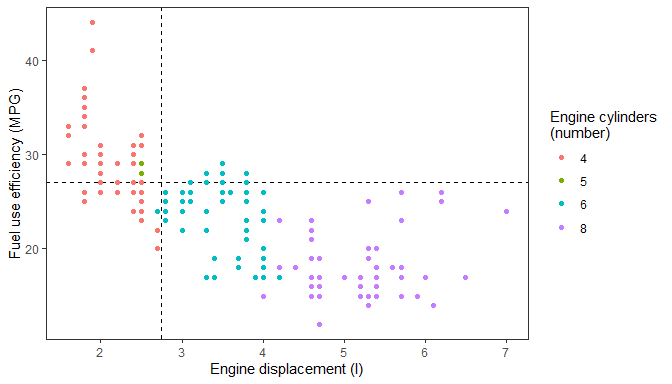### geom_text_s() and geom_point_s()

With package ‘ggrepel’ we have become used to easily add text and labels linked to the labelled observations or points by a line segment or arrow. However, sometimes a plot has few text labels and repulsion is unnecessary. In such cases nudging or dodging may be enough, or even preferable so as to achieve consistency.

We can achieve this with geom_text_s() (formerly named geom_text_linked()) together with a suitable position function that retains the original x and y coordinates of the point. We currently provide three different nudge functions described in a later section. One of them is used by default providing the same default behaviour as geom_text() from ‘ggplot2’ but drawing linking segments if nudging is set to a value different to the default of zero. The equivalent for points connected with segments is geom_point_s().

my.cars <- mtcars[c(TRUE, FALSE, FALSE, FALSE), ]
my.cars$name <- rownames(my.cars) my.cars <- my.cars[order(my.cars$wt), ]
ggplot(my.cars, aes(wt, mpg, label = name)) +
geom_point() +
geom_text_s(aes(colour = factor(cyl)),
angle = 90,
hjust = 1.04, nudge_y = -1,
nudge_x = c(0, 0, -0.05, +0.05, 0, 0 ,0, 0),
arrow = arrow(length = grid::unit(1.5, "mm"))) +
scale_colour_discrete(l = 40) +
expand_limits(y = 0)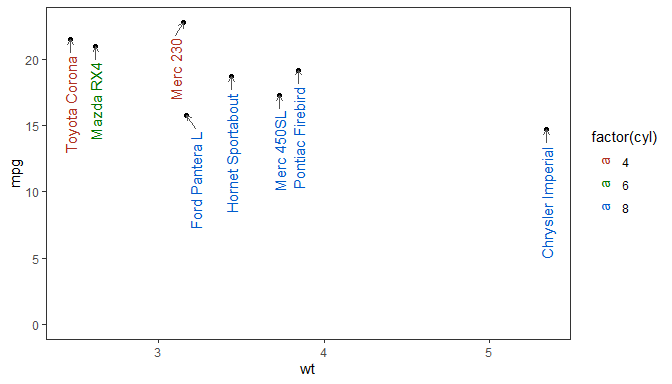### Normalised Parent Coordinates

R’s ‘grid’ package defines several units that can be used to describe the locations of plot elements. In ‘ggplot2’ the x and y aesthetics are directly mapped to "native" or data units. For consistent location of annotations with respect to the plotting area we need to rely on "npc" which are expressed relative to the size of the grid viewport. The plotting area in a ggplot is implemented as a ‘grid’ viewport and support for "npc" coordinates is relatively easy to implement.

To support "npc" positions we have implemented scales for two new (pseudo) aesthetics, npcx and npcy. These are very simple continuous scales which do not support any transformation or changes to their limits, both of which would be meaningless for "npc" units. Variables mapped to these aesthetics can be either numerical with values in the range zero to one or character. A limited set of strings are recognised and converted to "npc" units: "bottom", "center", "top", "left", "middle", "right" ("centre" is a synonym for "center").

To make these scales useful we need also to define geometries that use these new aesthetics. Package ‘ggpp’ currently provides geom_text_npc(), geom_label_npc(), geom_table_npc(), geom_plot_npc() and geom_grob_npc().

As is the case for geom_text() and geom_label() from package ‘ggplot2’, "bottom", "center", "top", "left", "middle", "right", plus "inward" and "outward" can be used, as well as numeric values, to control the justification. Justification defaults to "inward" in the geometries described here.

While the usual x and y aesthetics are used whenever the positions of plot elements represent data values, these new scales and geometries are useful only for annotations, i.e., in those cases when we want plot elements at specific positions within the plotting area irrespective of the ranges of the data mapped to the x and y aesthetics. When writing scripts or functions that may be applied to different data sets these new aesthetics help by keeping the code concise and reusable. These geometries are used by default by several of the statistics described in later sections and those defined in package ‘ggpmisc’.

As an example let’s imagine that we want to add the structure of a metabolite to a plot. Its position has nothing to do with the data mapped to x and y, so it is conceptually better to use "npc" coordinates. The big practical advantage is that this also allows to keep this part of the plot definition independent of the data being plotted, giving a major advantage in the case of plots with facets with free scale limits. This example can be easily adapted to geom_plot_npc() where a list of ggplots is mapped to label, and to geom_table_npc() where a list of data frame is mapped to label.

We produce the example plot by first constructing a tibble to contain the grob and the coordinate data, and then map these variables to aesthetics using aes(). In the example the tibble has a single row, but this is not a requirement. In this respect these geoms behave as normal geoms, with facets also supported.

file.name <-
system.file("extdata", "Robinin.png",
package = "ggpp", mustWork = TRUE)

set.seed(123456)
data.tb <- tibble(x = 1:20, y = (1:20) + rnorm(20, 0, 10))

flavo.tb <- tibble(x = 0.02,
y = 0.95,
width = 1/2,
height = 1/4,
grob = list(grid::rasterGrob(image = Robinin)))

ggplot(data.tb, aes(x, y)) +
geom_grob_npc(data = flavo.tb,
aes(label = grob, npcx = x, npcy = y,
vp.width = width, vp.height = height)) +
geom_point() +
expand_limits(y = 55, x = 0)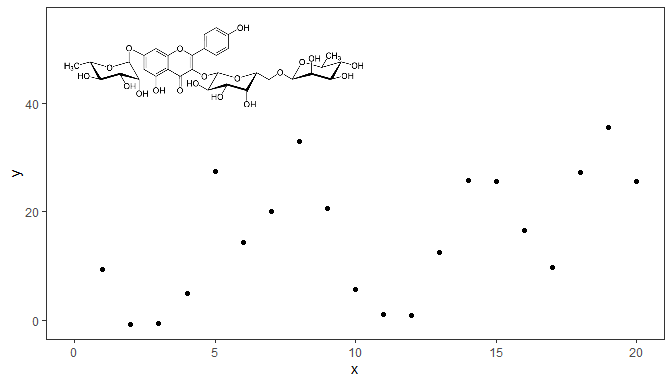Alternatively, we can pass constant values to geom_grob_npc() to obtain the same plot. This approach can be handy in simple cases.

ggplot(data.tb, aes(x, y)) +
geom_grob_npc(label = list(grid::rasterGrob(image = Robinin, width = 1)),
npcx = 0.02, npcy = 0.95,
vp.width = 1/2, vp.height = 1/4) +
geom_point() +
expand_limits(y = 55, x = 0)

We can also use annotate() if the annotation should be the same for all panels, or if we have a single figure panel. In this case there is no need to wrap a single grob in a list.

ggplot(data.tb, aes(x, y)) +
annotate("grob_npc", label = grid::rasterGrob(image = Robinin, width = 1),
npcx = 0.02, npcy = 0.95, vp.width = 1/2, vp.height = 1/4) +
geom_point() +
expand_limits(y = 55, x = 0)

Two additional geometries are based on existing ‘ggplot2’ geometries. They are based on geom_text() and geom_label(). We give an example using geom_text_npc() to produce a “classic” labelling for facets matching the style of theme_classic() and traditional scientific journals’ design.

corner_letters.tb <- tibble(label = LETTERS[1:4],
x = "left",
y = "top",
cyl = c(4,5,6,8))
ggplot(mpg, aes(displ, hwy)) +
geom_point() +
facet_wrap(~cyl, scales = "free") +
geom_text_npc(data = corner_letters.tb,
aes(npcx = x, npcy = y, label = label)) +
theme_classic() +
theme(strip.background = element_blank(),
strip.text.x = element_blank())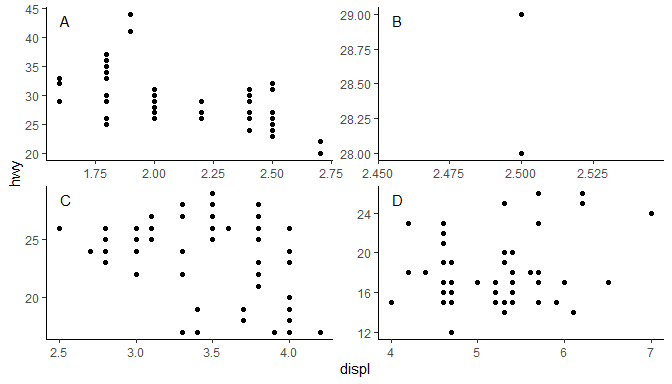### Marginal markings

‘ggplot2’ provides geom_rug(), geom_vline() and geom_hline(). Rug plots are intended to be used to represent distributions along the margins of plot. geom_vline() and geom_hline()are normally used to separate regions in a plot or to highlight important values along the x or y axis. When creating plots it is sometimes useful to put small marks along the axes, just inside the plotting area, similar to those in a rug plot, but like geom_vline() and geom_hline() in their purpose.

Three geometries provide such markers: geom_margin_point(), geom_margin_arrow(), and geom_margin_grob(). They behave similarly to geom_vline() and geom_hline() and their positions are determined also by the xintercept and yintercept aesthetics.

In the example below we indicate the group medians along the x axis with filled triangles.

data.tb <- mpg %>%
group_by(cyl) %>%
summarise(hwy = median(hwy), displ = median(displ))
ggplot(mpg, aes(displ, hwy, colour = factor(cyl))) +
geom_x_margin_point(data = data.tb,
aes(xintercept = displ, fill = factor(cyl))) +
expand_limits(y = 10) +
geom_point()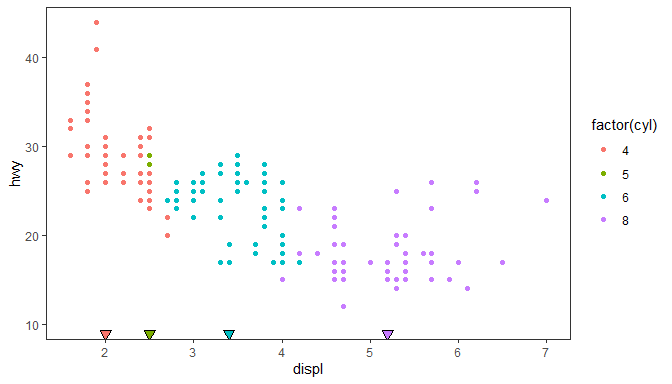## Statistics

### stat_centroid()

It can be useful to mark the centroid of a group of observations with a point or with a label. By default stat_centroid() applies function mean_se() to both x and y by group. If the value mapped to an aesthetics across rows within each group is unique this value is copied to the returned data.

ggplot(mpg, aes(displ, hwy, colour = factor(cyl))) +
geom_point(alpha = 0.33) +
stat_centroid(shape = "cross", size = 4)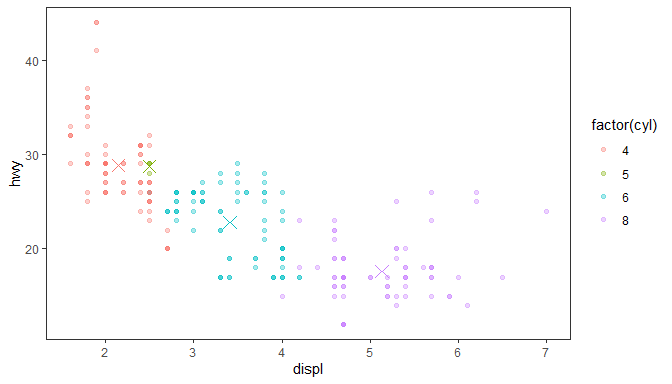Other functions can be passed to this statistic as long as they return a single value that can be mapped to the x and y aesthetics (numeric, time or a factor).

ggplot(mpg, aes(displ, hwy, colour = factor(cyl))) +
geom_point(alpha = 0.33) +
stat_centroid(shape = "cross", size = 4, .fun = median)

The very similar stat_summary_xy() accepts different functions for x and y.

This statistic automates the annotation of plots with number of observations, either by quadrant, by pairs of quadrants or the four quadrants taken together (whole plotting area). Its companion geometry, geom_quadrant_lines() is used in the examples to highlight the quadrants.

We generate some artificial data.

set.seed(4321)
# generate artificial data
x <- -99:100
y <- x + rnorm(length(x), mean = 0, sd = abs(x))
my.data <- data.frame(x,
y,
group = c("A", "B"))

Using defaults except for color.

ggplot(my.data, aes(x, y)) +
geom_point() +
expand_limits(y = c(-250, 250))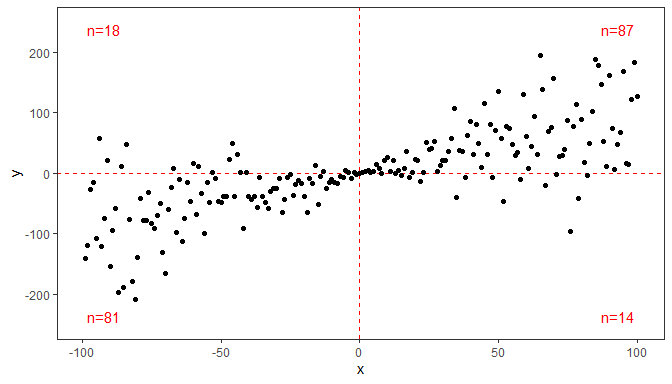Pooling quadrants along the x-axis. (pool.along = "y" pools along y.)

ggplot(my.data, aes(x, y)) +
geom_quadrant_lines(colour = "red", pool.along = "x") +
stat_quadrant_counts(colour = "red", pool.along = "x") +
geom_point()

Manual positioning of the text annotations and pooling of all four quadrants, and overriding the default formatting for the label.

ggplot(my.data, aes(x, y)) +
geom_point() +
aes(label = sprintf("%i observations", stat(count))))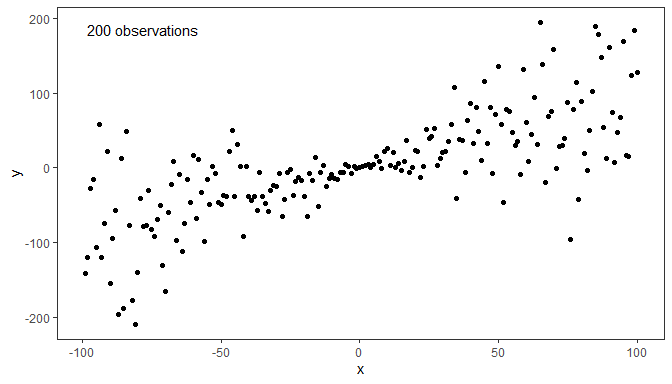ggplot(my.data, aes(x, y)) +
geom_point()

Using facets, even with free scale limits, the labels are placed consistently. This achieved by the default use of geom_text_npc() or as shown below by use of geom_label_npc(). We expand the y limits to ensure that no observations are occluded by the labels.

ggplot(my.data, aes(x, y, colour = group)) +
geom_point() +
expand_limits(y = c(-260, 260)) +
facet_wrap(~group)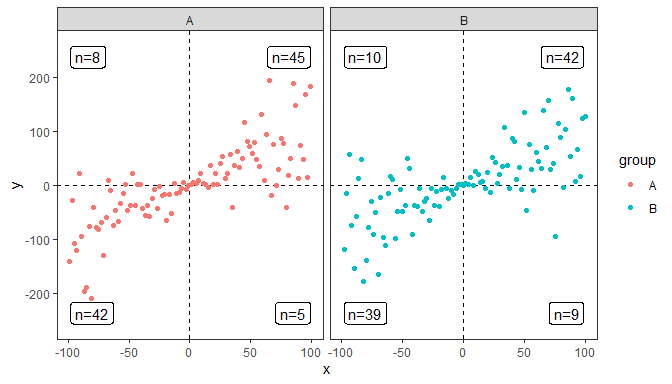### stat_apply_group

This statistic applies functions to x and y data. The function(s) supplied as argument are expected to return a numeric vector. Both functions should return vectors of the same length. When possible it is usually better to apply functions through mappings using aes() when they are independent of grouping, or to use a transformation for the scale. However, when grouping is important, these statistics make it possible to avoid pre-computation of the data for a layer.

Here we plot the difference in trunck circumference between dates for each tree. (As the vector returned by diff() is one element shorter than its input, we delete the first value of x in .fun.x.).

ggplot(Orange, aes(age, circumference, colour = Tree)) +
stat_apply_group(.fun.x = function(x) {x[-1L]},
.fun.y = diff)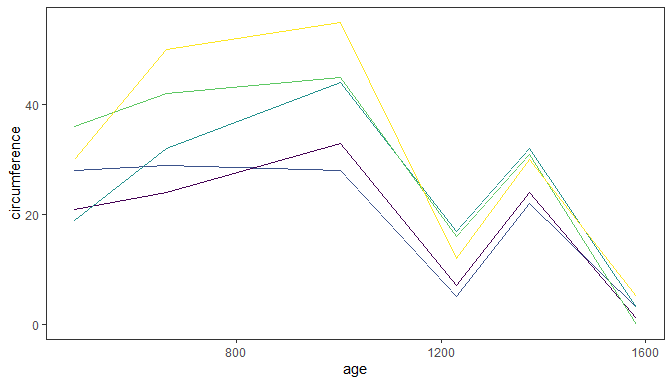### stat_dens2d_labels() and stat_dens2d_filter()

These stats had their origin in an enhancement suggestion for ‘ggrepel’ from Hadley Wickham and discussion with Kamil Slowikowski (ggrepel’s author) and others. In fact the code is based on code Kamil gave during the discussion, but simplified and taking a few further ideas from ggplot::stat_dens2d.

Warning! Which observations are selected by the algorithm used, based on MASS:kde2d, depends strongly on the values of parameters h and n. You may need to alter the defaults by passing explicit arguments to these stats. Beware, though, that what are good values, may depend on individual data sets even if they include the same number of observations. For the selection of observations to work cleanly, the argument for n must create a dense grid. Much larger values of n than in the examples in the documentation of MASS::kde2d and ggplot2::stat_dens2d will be needed in most cases.

Some random data with random labels.

random_string <- function(len = 3) {
paste(sample(letters, len, replace = TRUE), collapse = "")
}

# Make random data.
set.seed(1001)
d <- tibble::tibble(
x = rnorm(100),
y = rnorm(100),
group = rep(c("A", "B"), c(50, 50)),
lab = replicate(100, { random_string() })
)

The stat stat_dens2d_filter filters observations, in other words passes to the geom a subset of the data received as input. The default argument for geom is "point".

Using defaults except for the color aesthetic. Highlight 1/4 of observations from lowest density areas of the plot panel.

ggplot(data = d, aes(x, y)) +
geom_point() +
stat_dens2d_filter(keep.fraction = 1/4, colour = "red")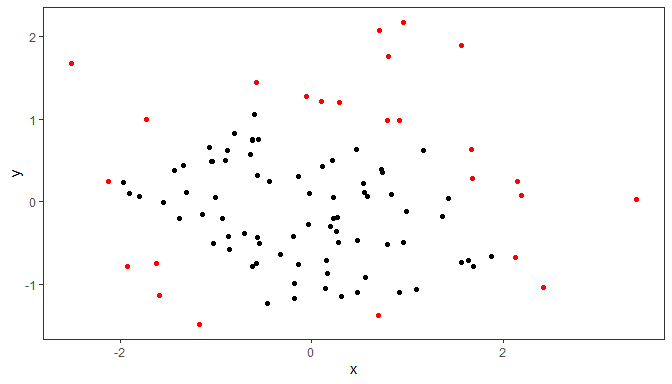Keep at most 50 observations.

ggplot(data = d, aes(x, y)) +
geom_point() +
stat_dens2d_filter(keep.fraction = 1/4, keep.number = 50, colour = "red")

Keep always 50 observations.

ggplot(data = d, aes(x, y)) +
geom_point() +
stat_dens2d_filter(keep.fraction = 1, keep.number = 50, colour = "red")

Highlighting 1/4 of the observations by under-plotting with larger black points.

ggplot(data = d, aes(x, y, colour = group)) +
stat_dens2d_filter(keep.fraction = 0.25,
size = 3,
colour = "black") +
geom_point()

A different way of highlighting 1/4 of the observations, using over-plotting with a ‘hollow’ shape. We also shift one group with respect to the other.

ggplot(data = d, aes(x + rep(c(-2,2), rep(50,2)),
y, colour = group)) +
geom_point() +
stat_dens2d_filter(shape = 1, size = 3,
keep.fraction = 0.25)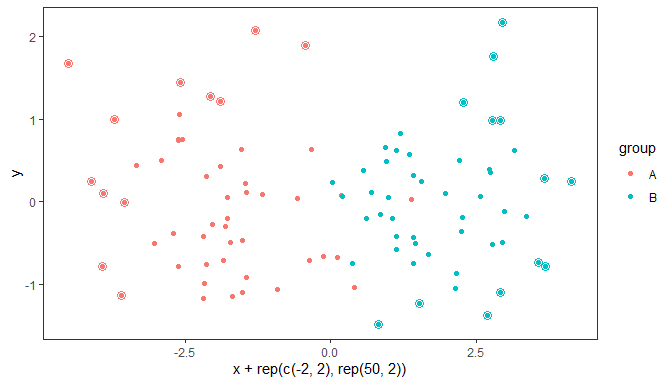Highlight 1/4 of observations from lowest density areas of the plot, with density considered separately for each individual group. In this case grouping is based on the color aesthetic.

ggplot(data = d, aes(x + rep(c(-2,2), rep(50,2)),
y, colour = group)) +
geom_point() +
stat_dens2d_filter_g(shape = 1, size = 3,
keep.fraction = 0.25)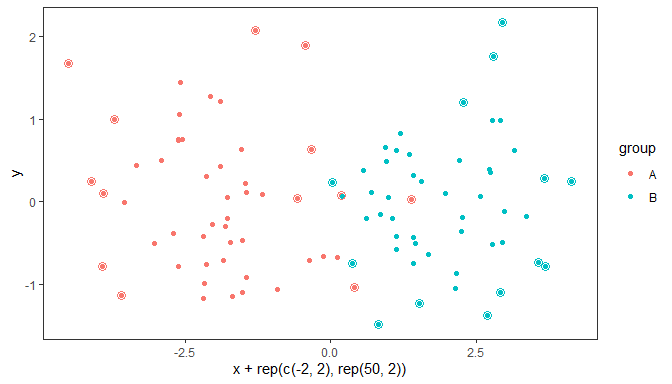The stat stat_dens1d_labels replaces the values of the label (aesthetic) variable in data based on density of observations along the x or y axis in the plot panel. The replacement is given by the argument passed to label.fill, which can be a character string or a function accepting a character string as argument and returning also a character string.

The default value for geom is "text". The default value of label.fill is "" which results in empty labels, while using NA as fill label results in observations being omitted. Using NA as label.fill is similar to using stat_dens2d_filter as long as the geom used requires a label aesthetic.

Label 1/10 of observations from lowest density areas of the plot panels.

ggplot(data = d, aes(x, y, label = lab, colour = group)) +
stat_dens2d_labels(keep.fraction = 1/10,
hjust = "outward", vjust = "outward") +
geom_point()

Using the geoms from package ‘ggrepel’ avoids clashes among labels or on top of data points. This works with versions 0.6.0 and newer of ‘ggrepel’. One example with geom_text_repel follows.

ggplot(data = d, aes(x, y, label = lab, colour = group)) +
geom_point() +
stat_dens2d_labels(geom = "text_repel",
keep.fraction = 0.45)

With geom_label_repel one usually needs to use a smaller value for keep.fracton, or a smaller size, as labels use more space on the plot than the test alone.

Additional arguments can be used to change the angle and position of the text, but may give unexpected output when labels are long as the repulsion algorithm “sees” always a rectangular bounding box that is not rotated. With short labels or angles that are multiples of 90 degrees, there is no such problem. Please, see the documentation for ggrepel::geom_text_repel and ggrepel::geom_label_repel for the various ways in which both repulsion and formatting of the labels can be adjusted.

Using NA as argument to label.fill makes the observations with labels set to NA incomplete, and such rows in data are skipped when rendering the plot, before the repulsion algorithm is active. This can lead to overlap between text and points corresponding to unlabelled observations. Whether points are occluded depends on the order of layers and transparency, the occlusion can remain easily unnoticed with geom_label and geom_label_repel. We keep geom_point as the topmost layer to ensure that all observations are visible.

ggplot(data = d, aes(x, y, label = lab, colour = group)) +
stat_dens2d_labels(geom = "label_repel",
keep.fraction = 0.2,
label.fill = NA) +
geom_point()

### stat_dens1d_labels() and stat_dens1d_filter()

These stats are similar to stat_dens2d_labels() and stat_dens2d_filter() but compute the density in a single dimension, either the x or y aesthetics.

Warning! Which observations are selected by the algorithm used, based on stats::density, depends strongly on the values of parameters bw, adjust and kernel. You may need to alter the defaults by passing explicit arguments. Beware that what are good values, may depend on individual data sets even if they include the same number of observations. For the selection of observations to work cleanly, the argument for n must large enough to generate a dense grid or the bandwidth may need to be increased by passing a number > 1 as argument. Increasing the bandwidth makes the empirical density function smoother, and the selection of points less dependent on immediate neighbours.

We use the same data as in the previous sections.

random_string <- function(len = 6) {
paste(sample(letters, len, replace = TRUE), collapse = "")
}

# Make random data.
set.seed(1001)
d <- tibble::tibble(
x = rnorm(100),
y = rnorm(100),
group = rep(c("A", "B"), c(50, 50)),
lab = replicate(100, { random_string() })
)

The stat stat_dens1d_filter filters observations, in other words passes to the geom a subset of the data received as input. The default value for geom is "point" and the default orientation is "x".

Using defaults except for the color aesthetic, we highlight 1/4 of observations from lowest density region along the x axis of the plot panel.

ggplot(data = d, aes(x, y)) +
geom_point() +
stat_dens1d_filter(keep.fraction = 0.25,
colour = "red")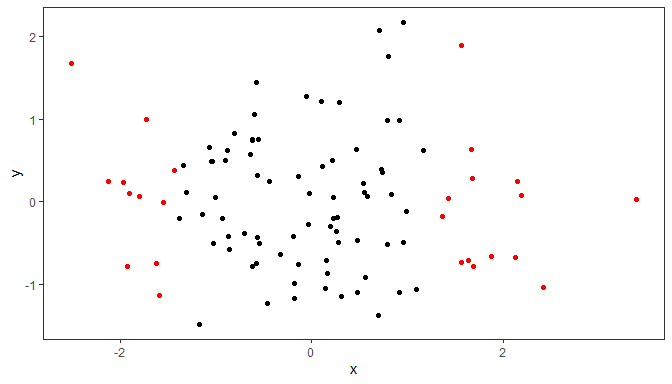We repeat the example above, we highlight 1/4 of observations, but now from lowest density region along the y axis of the plot panel.

ggplot(data = d, aes(x, y)) +
geom_point() +
stat_dens1d_filter(keep.fraction = 0.25,
colour = "red",
orientation = "y")

In other respects than orientation and the parameters passed internally to stats::density() the examples given earlier for stat_dens2d_filter() also apply.

The stat stat_dens1d_labels replaces the values of the label (aesthetic) variable in data based on density of observations along the x or y axis in the plot panel. The replacement is given by the argument passed to label.fill, which can be a character string or a function accepting a character string as argument and returning also a character string.

The default value for geom is "text". The default value of label.fill is "" which results in empty labels, while using NA as fill label results in observations being omitted. Using NA as label.fill is similar to using stat_dens2d_filter as long as the geom used requires a label aesthetic.

Label 1/10 of observations from lowest density regions along x in the plot panel. Normally a repulsive geom is most useful.

ggplot(data = d, aes(x, y, label = lab, colour = group)) +
geom_point() +
stat_dens1d_labels(geom = "text_repel")

Similarly along the y axis.

ggplot(data = d, aes(x, y, label = lab, colour = group)) +
geom_point() +
stat_dens1d_labels(geom = "text_repel", orientation = "y")

In other respects than orientation and the parameters passed internally to stats::density() the examples given earlier for stat_dens2d_labels() also apply.

## Simple position functions

Nudging shifts deterministically the coordinates giving an x and/or y position and also expands the limits of the corresponding scales to match. By default in ‘ggplot2’ geometries and position functions no nudging is applied.

Function position_nudge() from package ‘ggplot2’ simply applies the nudge, or x and/or y shifts based directly on the values passed to its parameters x and y. Passing arguments to the nudge_x and/or nudge_y parameters of a geometry has the same effect as these values are passed to position_nudge(). Geometries also have a position parameter to which we can pass an expression based on a position function which opens the door to more elaborate approaches to nudging.

A new variation on simple nudge is provided by function position_nudge_to(), which accepts the desired nudged final coordinates directly instead of as a shift.

We can do better than simply shifting all data to the same extent and direction or to a fixed position. For example by nudging away from a focal point, a line or a curve. In position_nudge_center() and position_nudge_line() described below, this reference alters only the direction (angle) along which nudge is applied but not the extent of the shift. Advanced nudging works very well, but only for some patterns of observations and may require manual adjustment of positions, repulsion is more generally applicable but like jitter is aleatory. Combining nudging and repulsion we can make repulsion more predictable with little loss of its applicability.

These functions can be used with any geometry but if segments joining the labels to the points are desired, ggrepel::geom_text_repel() or ggrepel::geom_label_repel(), possibly setting max.iter = 0 if no repulsion is desired. Several of the functions defined in ‘ggpp’ even if not supporting repulsion, can plot connecting segments. Please see the documentation for these geometries for the details. Drawing of segments or arrows is made possible by storing in data both the nudged and original x and y coordinates. This is made possible by coordinated development of packages ‘ggpp’ and ‘ggrepel’ and agreement on a naming convention for storing the original position.

### position_nudge_keep() and friends

Function position_nudge_keep() is like ggplot2::position_nudge() but keeps (stores) the original x and y coordinates; position_nudge_keep() can be used interchangeably with ggplot2::position_nudge() with other geometries. Although possibly only occasionally needed, position_jitter_keep() , position_dodge_keep() and position_stack_keep() are also made available for completeness.

set.seed(84532)
df <- data.frame(
x = rnorm(20),
y = rnorm(20, 2, 2),
l = paste("label:", letters[1:20])
)

When used together with geom_text_s() or geom_label_s() segments between a nudged and/or repelled label and the original position (here indicated by a point) are drawn.

ggplot(df, aes(x, y, label = l)) +
geom_point() +
geom_text_s(position = position_nudge_keep(x = 0.1),
hjust = "left") +
expand_limits(x = 2.5)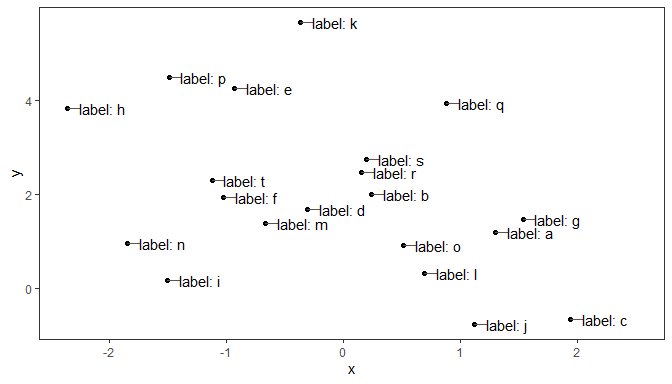We can supress the drawing of segments.

ggplot(df, aes(x, y, label = l)) +
geom_point() +
geom_text_s(position = position_nudge_keep(x = 0.1),
hjust = "left", add.segments = FALSE) +
expand_limits(x = 2.5)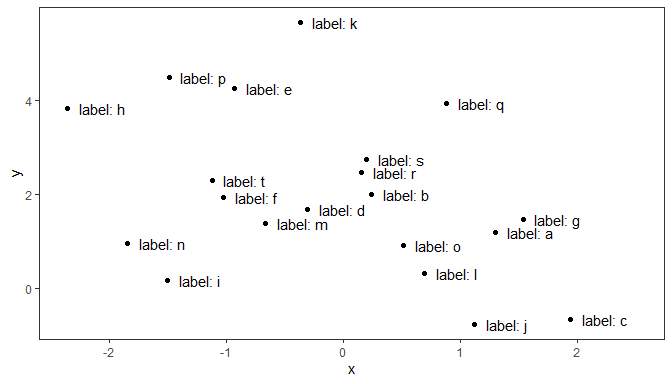With position_nudge() used together with geom_text_repel() or geom_label_repel() segments connecting a nudged and/or repelled label and the original position (here indicated by a point) are, by default, not drawn unless they are longer than a minimum.

ggplot(df, aes(x, y, label = l)) +
geom_point() +
geom_text_repel(position = position_nudge(x = 0.3),
min.segment.length = 0,
max.iter = 0)

With geom_text_repel() or geom_label_repel() we can force drawing of segments irespective of their length if we pass min.segment.length = 0 in the call.

ggplot(df, aes(x, y, label = l)) +
geom_point() +
geom_text_repel(position = position_nudge_keep(x = 0.3),
min.segment.length = 0, max.iter = 0)

position_nudge_keep() and all other position functions described below save the original positions in data in columns x_orig and y_orig and the shifted positions in columns x and y. Because of this, they can be used together with any ‘ggplot2’ geometry, even though these geometries will ignore the stored original positions.

ggplot(df, aes(x, y, label = l)) +
geom_point() +
geom_text(position = position_nudge(x = 0.3))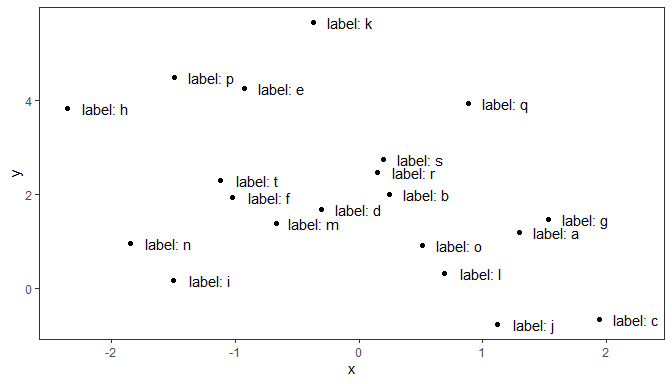### position_nudge_to()

Function position_nudge_to() nudges to a given position instead of using the same shift for each observation. Can be used to align labels for points that are not themselves aligned. By left-justifying the label text we ensure the alignment of labels differing in width.

ggplot(df, aes(x, y, label = ifelse(x < 1, "", l) )) +
geom_point() +
geom_text_s(position = position_nudge_to(y = 2.3),
segment.color = "red",
arrow = arrow(length = unit(0.015, "npc")),
angle = 90,
hjust = "left") +
expand_limits(x = 3)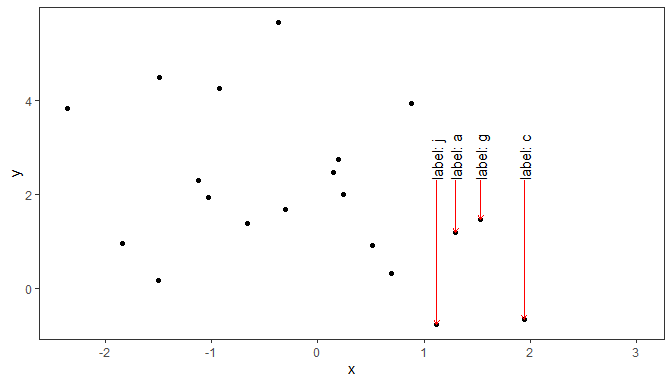We here the pass a vector of length one as argument for y, but x and y also accept vectors of the same length as rows has data. In other words, it allows manual positioning of text and labels.

ggplot(df, aes(x, y, label = l)) +
geom_point(color = "red", size = 3) +
geom_text_repel(position = position_nudge_to(y = -2.5),
size = 3,
color = "red",
angle = 90,
hjust = 0,
segment.color = NA,
direction = "x") +
geom_rug(sides = "b", length = unit(0.02, "npc"), color = "red")

### position_nudge_center()

Function position_nudge_center() can nudge radially away from a focal point if both x and y are passed as arguments, or towards opposite sides of a boundary vertical or horizontal virtual line if only one of x or y is passed an argument. By default, the “center” is the centroid computed using mean(), but other functions or numeric values can be passed to override it. When data are sparse, such nudging may be effective in avoiding label overlaps, and achieving a visually pleasing positioning.

In all cases nudging shifts the coordinates giving an x and/or y position and also expands the limits of the corresponding scales to include the nudged coordinate values.

ggplot(df, aes(x, y, label = l)) +
geom_point() +
geom_text_repel(position =
position_nudge_center(x = 0.3, center_x = 0),
min.segment.length = 0, max.iter = 0)

By default, split is away or towards the mean(). Here we allow repulsion to separate the labels (compare with previous plot).

ggplot(df, aes(x, y, label = l)) +
geom_point() +
geom_text_repel(position =
position_nudge_center(x = 0.3,
direction = "split"),
min.segment.length = 0)

We set a different split point as a constant value.

ggplot(df, aes(x, y, label = l)) +
geom_point() +
geom_text_repel(position =
position_nudge_center(x = 0.3,
center_x = 1,
direction = "split"),
min.segment.length = 0)

We set a different split point as the value computed by a function function, by name.

ggplot(df, aes(x, y, label = l)) +
geom_point() +
geom_text_repel(position =
position_nudge_center(x = 0.3,
center_x = median,
direction = "split"),
min.segment.length = 0)

We set a different split point as the value computed by an anonymous function function. Here que split on the first quartile along x.

ggplot(df, aes(x, y, label = l)) +
geom_point() +
geom_text_repel(position =
position_nudge_center(x = 0.3,
center_x = function(x) {
quantile(x,
probs = 1/4,
names = FALSE)
},
direction = "split"),
min.segment.length = 0)

The labels can be rotated as long as the geometry used supports this.

ggplot(df, aes(x, y, label = l)) +
geom_point() +
geom_text_repel(angle = 90,
position =
position_nudge_center(y = 0.1,
direction = "split"))

By requesting nudging along x and y and setting direction = "split" nudging is applied according to the quadrants centred on the centroid of the data.

ggplot(df, aes(x, y, label = l)) +
geom_point() +
geom_text_repel(position =
position_nudge_center(x = 0.1,
y = 0.15,
direction = "split"))

With direction = "radial", the distance nudged away from the center is the same for all labels.

ggplot(df, aes(x, y, label = l)) +
geom_point() +
geom_text_repel(position =
position_nudge_center(x = 0.25,
y = 0.4,
min.segment.length = 0)

As shown above for direction = "split" we can set the coordinates of the centre by passing constant values as in this example or functions (possibly different for x and y). We can also set the justification the text labels although repulsion usually works best with labels justified at the centre, which is the default.

ggplot(df, aes(x, y, label = l)) +
geom_point() +
geom_text_repel(position =
position_nudge_center(x = 0.15,
y = 0.3,
center_x = 0,
center_y = 0,
min.segment.length = 0,
hjust = "outward", vjust = "outward")

Nudging along one axis, here x, and setting the repulsion direction along the other axis, here y, tends to give a pleasent arrangement of labels.

ggplot(df, aes(x, y, label = l)) +
geom_point() +
geom_text_repel(position =
position_nudge_center(x = 0.2,
center_x = 0,
direction = "split"),
aes(hjust = ifelse(x < 0, 1, 0)),
direction = "y",
min.segment.length = 0) +
expand_limits(x = c(-3, 3))

### position_nudge_line()

Function position_nudge_line() nudges away from a line, which can be a user supplied straight line as well as a smooth spline or a polynomial fitted to the observations themselves. The nudging is away and perpendicular to the local slope of the straight or curved line. It relies on the same assumptions as linear regression, assuming that x values are not subject to error. This in most cases prevents labels from overlaping a curve fitted to the data, even if not exactly based on the same model fit. When observations are sparse, this may be enough to obtain a nice arrangement of data labels.

set.seed(16532)
df <- data.frame(
x = -10:10,
y = (-10:10)^2,
yy = (-10:10)^2 + rnorm(21, 0, 4),
yyy = (-10:10) + rnorm(21, 0, 4),
l = letters[1:21]
)

The first, simple example shows that position_nudge_line() has shifted the direction of the nudging based on the alignment of the observations along a line. One could, of course, have in this case passed suitable values as arguments to x and y using position_nudge() from package ‘ggplot2’. However, position_nudge_line() will work without change irrespective of the slope or intercept along which the observations fall.

ggplot(df, aes(x, 2 * x, label = l)) +
geom_point() +
geom_abline(intercept = 0, slope = 2, linetype = "dotted") +
geom_text(position = position_nudge_line(x = -0.5, y = -0.8))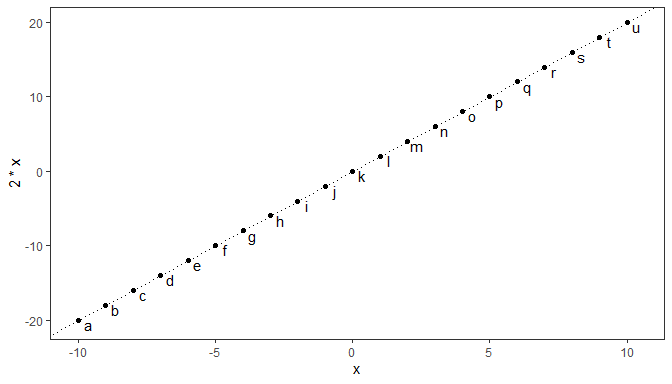With observations with high variation in y, a linear model fit may need to be used. In this case fitted twice, once in stat_smooth() and once in position_nudge_line().

ggplot(subset(df, x >= 0), aes(x, yyy)) +
geom_point() +
stat_smooth(method = "lm") +
geom_text(aes(label = l),
vjust = "center", hjust = "center",
position = position_nudge_line(x = 0, y = 1.2,
method = "lm",
direction = "split"))
## geom_smooth() using formula 'y ~ x'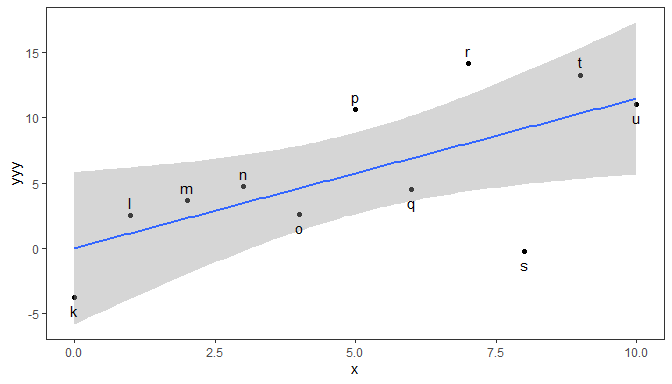With lower variation in y, we can pass to line_nudge a multiplier to keep labels outside of the confidence band.

ggplot(subset(df, x >= 0), aes(y, yy)) +
geom_point() +
stat_smooth(method = "lm", formula = y ~ x) +
geom_text(aes(label = l),
position = position_nudge_line(method = "lm",
x = 3, y = 3,
line_nudge = 2.5,
direction = "split"))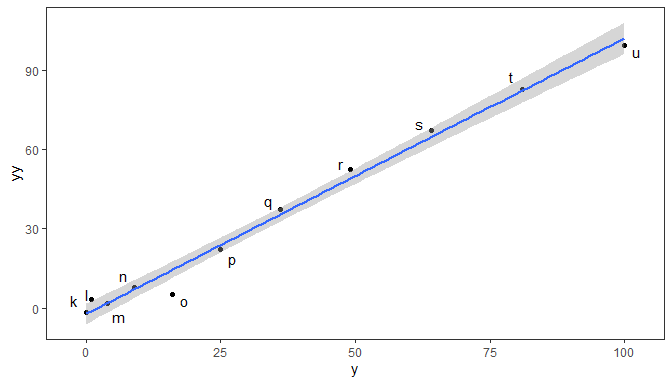If we want the nudging based on an arbitrary straight line not computed from data, we can pass the intercept and slope in a numeric vector of length two as an argument to parameter abline.

ggplot(subset(df, x >= 0), aes(y, yy)) +
geom_point() +
geom_abline(intercept = 0, slope = 1, linetype = "dotted") +
geom_text(aes(label = l),
position = position_nudge_line(abline = c(0, 1),
x = 3, y = 3,
direction = "split"))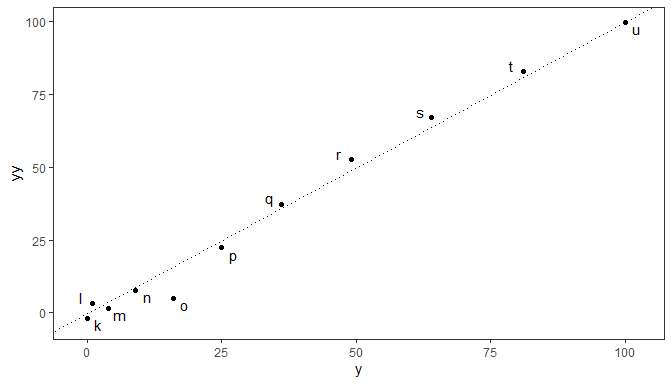With observations that follow exactly a simple curve the defaults work well to automate the nudging of individual data labels away from the implicit curve. Positive values as arguments to x and y correspond to above and inside the curve. One could, of course, pass also in this case suitable values as arguments to x and y using position_nudge() from package ‘ggplot2’, but these arguments would need to be vectors with different nudge values for each observation.

ggplot(df, aes(x, y, label = l)) +
geom_point() +
geom_line(linetype = "dotted") +
geom_text(position = position_nudge_line(x = 0.6, y = 6))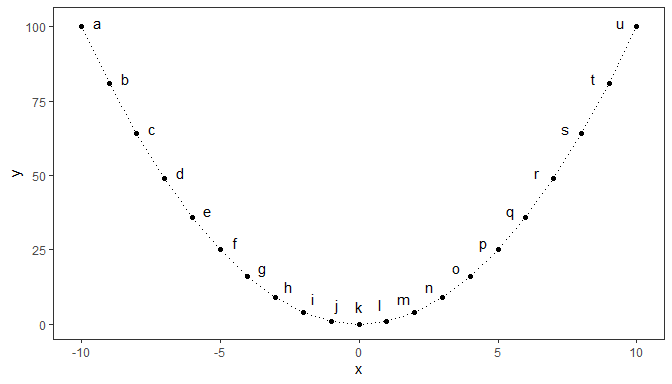Negative values passed as arguments to x and y correspond to labels below and outside the curve.

ggplot(df, aes(x, y, label = l)) +
geom_point() +
geom_line(linetype = "dotted") +
geom_text(position = position_nudge_line(x = -0.6, y = -6))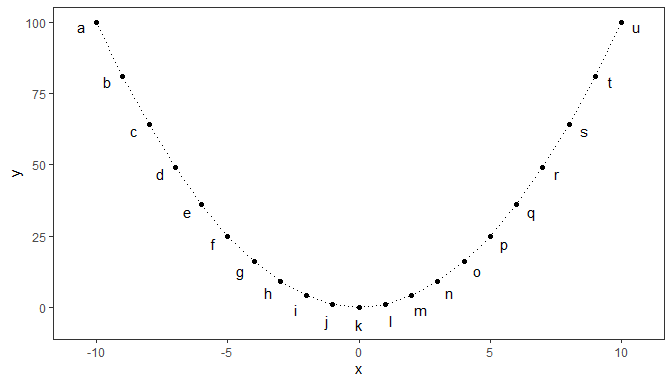When the observations include random variation along y, it is important that the smoother used for the line added to a plot and that passed to position_nudge_line() are similar. By default stat_smooth() uses "loess" and position_nudge_line() with method "spline", smooth.sline(), which are a good enough match.

ggplot(df, aes(x, yy, label = l)) +
geom_point() +
stat_smooth() +
geom_text(aes(y = yy, label = l),
position = position_nudge_line(x = 0.6,
y = 6,
direction = "split"))
## geom_smooth() using method = 'loess' and formula 'y ~ x'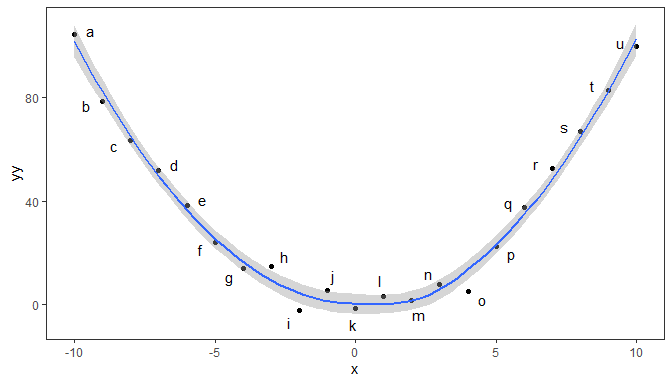We can use other geometries.

ggplot(df, aes(x, yy, label = l)) +
geom_point() +
stat_smooth() +
geom_label_repel(aes(y = yy, label = paste("point", l)),
position = position_nudge_line(x = 0.6,
y = 6,
direction = "split"),
min.segment.length = 0)

When fitting a polynomial, "lm" should be the argument passed to method and a model formula preferably based on poly(), setting raw = TRUE, as argument to formula. Currently no other methods are implemented in position_nudge_line().

ggplot(df, aes(x, yy, label = l)) +
geom_point() +
stat_smooth(method = "lm", formula = y ~ poly(x, 2, raw = TRUE)) +
geom_text(aes(y = yy, label = l),
position = position_nudge_line(method = "lm",
x = 0.5,
y = 5,
formula = y ~ poly(x, 2, raw = TRUE),
direction = "split"))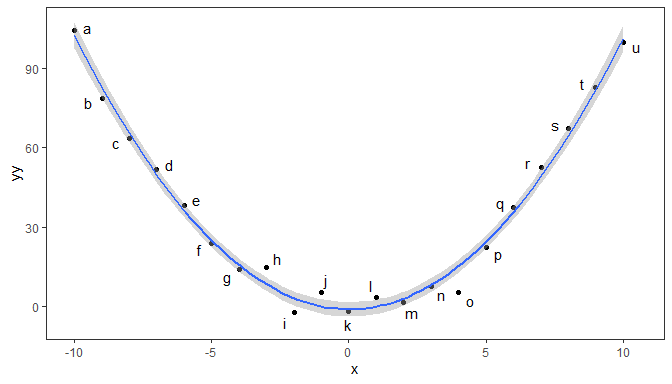Compared to pure repulsion adding nudging helps “direct” repulsion in the desired direction. Compare the two plots bellow using no nudging with the two above.

ggplot(df, aes(x, yy, label = l)) +
geom_point() +
stat_smooth(method = "lm", formula = y ~ poly(x, 2, raw = TRUE)) +
geom_text_repel(aes(y = yy, label = l),
min.segment.length = Inf)
ggplot(df, aes(x, yy, label = l)) +
geom_point() +
stat_smooth() +
geom_label_repel(aes(y = yy, label = paste("point", l)),
min.segment.length = 0)

Grouping is supported.

df <- data.frame(x = rep(1:10, 2),
y = c(1:10, 10:1),
group = rep(c("a", "b"), c(10, 10)),
l = "+")
ggplot(df, aes(x, y, label = l, color = group)) +
geom_line(linetype = "dotted") +
geom_text() +
geom_text(position = position_nudge_line(x = 0.25, y = 1)) +
geom_text(position = position_nudge_line(x = -0.25, y = -1)) +
coord_equal(ratio = 0.5)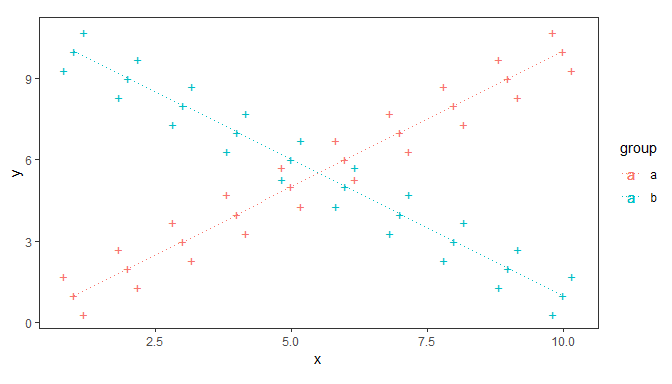One needs to ensure that grouping is in effect in the geoms with nudging.

ggplot(df, aes(x, y, label = l, color = group, group = group)) +
geom_line(linetype = "dotted") +
geom_text() +
geom_text(color = "red",
position = position_nudge_line(x = 1, y = 1)) +
geom_text(color = "blue",
position = position_nudge_line(x = -1, y = -1)) +
coord_equal()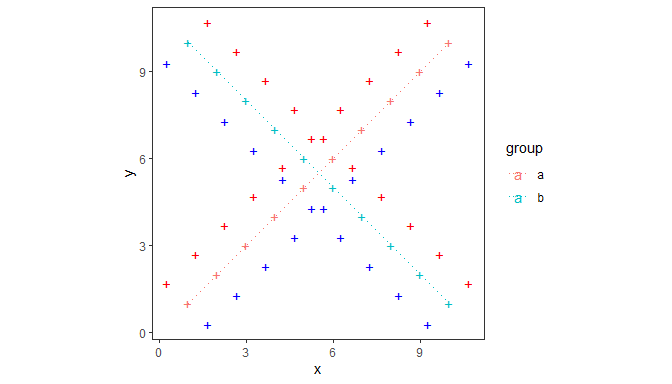Facets are also supported.

ggplot(df, aes(x, y, label = l)) +
geom_line(linetype = "dotted") +
geom_text() +
geom_text(position = position_nudge_line(x = 1, y = 1),
color = "red") +
geom_text(position = position_nudge_line(x = -1, y = -1),
color = "blue") +
facet_wrap(~group) +
coord_equal(ratio = 1)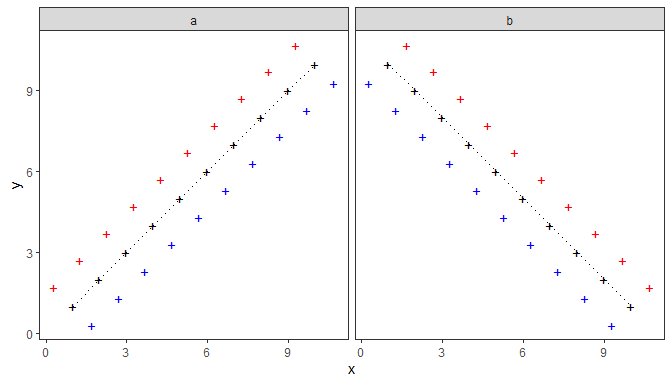## Combined position functions

Nudging text or labels in a dodged or stacked bar or column plot has not been easy in ‘ggplot2’. This is a rather frequent situation, although usually there are workarounds. Non-the-less this imposed some limitations on the design of plots. The examples below show the use of position that implement the functionality of more than one of the position functions available in ‘ggplot2’. Examples will be added here for the next release. Meanwhile, please, read the help page for each function. These pages contain examples.

## Appendix A: More on density filtering

We define a function to simplify the generation of random data sets based on different probability distributions.

make_data_tbl <- function(nrow = 100, rfun = rnorm, ...) {
if (nrow %% 2) {
nrow <- nrow + 1
}

set.seed(1001)

tibble::tibble(
x = rfun(nrow, ...),
y = rfun(nrow, ...),
group = rep(c("A", "B"), c(nrow / 2, nrow / 2))
)
}

In all the examples in this vignette we use colors to demonstrate which data points are selected, but any other suitable aesthetic and discrete scale can be used instead. With keep.sparse = FALSE we keep 1/3 of the observations in the denser region of the plot. Although here we first plot all data points and later overplot the selected ones this is not necessary.

ggplot(data = make_data_tbl(300), aes(x, y)) +
geom_point() +
stat_dens2d_filter(colour = "red",
keep.sparse = FALSE,
keep.fraction = 1/3)

Here we highlight the observations split in three group equal groups, each of a different density of observations.

ggplot(data = make_data_tbl(300), aes(x, y)) +
geom_point() +
stat_dens2d_filter(colour = "red",
keep.sparse = FALSE,
keep.fraction = 1/3)+
stat_dens2d_filter(colour = "blue",
keep.fraction = 1/3)

The algorithm seems to work well also with other distributions, in this example the uniform distribution.

ggplot(data = make_data_tbl(300, rfun = runif), aes(x, y)) +
geom_point() +
stat_dens2d_filter(colour = "red", keep.fraction = 1/2)

One example with the gamma distribution, which is asymmetric.

ggplot(data = make_data_tbl(300, rfun = rgamma, shape = 2),
aes(x, y)) +
geom_point() +
stat_dens2d_filter(colour = "red", keep.fraction = 1/3)

## Appendix B: try_tibble()

### Time series

Several different formats for storing time series data are used in R. Here we use in the examples objects of class ts but several other classes are supported as try.xts() is used internally. The first example is a quarterly series.

class(austres)
##  "ts"
austres.df <- try_tibble(austres)
class(austres.df)
##  "tbl_df"     "tbl"        "data.frame"
head(austres.df, 4)
## # A tibble: 4 x 2
##   time            x
##   <date>      <dbl>
## 1 1971-04-01 13067.
## 2 1971-07-01 13130.
## 3 1971-10-01 13198.
## 4 1972-01-01 13254.

The next chunk demonstrates that numeric times are expressed as decimal years in the returned data frame.

austres.df <- try_tibble(austres, as.numeric = TRUE)
head(austres.df, 4)
## # A tibble: 4 x 2
##    time      x
##   <dbl>  <dbl>
## 1 1971. 13067.
## 2 1971. 13130.
## 3 1972. 13198.
## 4 1972  13254.

This second example is for a series of yearly values.

class(lynx)
lynx.df <- try_tibble(lynx)
class(lynx.df)
head(lynx.df, 3)

Above there is a small rounding error of 1 s for these old dates. We can correct this by rounding to year.

lynx.df <- try_tibble(lynx, "year")
head(lynx.df, 3)
## # A tibble: 3 x 2
##   time           x
##   <date>     <dbl>
## 1 1822-01-01   269
## 2 1823-01-01   321
## 3 1824-01-01   585

In addition we can convert the POSIXct values into numeric values in calendar years plus a decimal fraction.

lynx_n.df <- try_tibble(lynx, "year", as.numeric = TRUE)
lapply(lynx_n.df, "class")
head(lynx_n.df, 3)

### Other classes

try_tibble() attempts to handle gracefully objects that are not time series.

try_tibble(1:5)
try_tibble(letters[1:5])
try_tibble(factor(letters[1:5]))
try_tibble(list(x = rep(1,5), y = 1:5))
try_tibble(data.frame(x = rep(1,5), y = 1:5))
try_tibble(matrix(1:10, ncol = 2))`